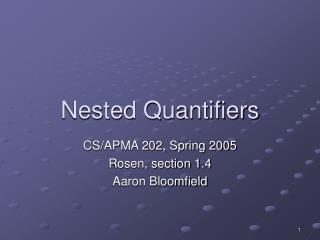DownloadDownload PresentationNested Quantifiers

# Nested Quantifiers

Download Presentation## Nested Quantifiers

- - - - - - - - - - - - - - - - - - - - - - - - - - - E N D - - - - - - - - - - - - - - - - - - - - - - - - - - -
##### Presentation Transcript

1. Nested Quantifiers CS/APMA 202, Spring 2005 Rosen, section 1.4 Aaron Bloomfield

2. Multiple quantifiers • You can have multiple quantifiers on a statement • xy P(x, y) • “For all x, there exists a y such that P(x,y)” • Example: xy (x+y == 0) • xy P(x,y) • There exists an x such that for all y P(x,y) is true” • xy (x*y == 0)

3. Order of quantifiers • xy and xy are not equivalent! • xy P(x,y) • P(x,y) = (x+y == 0) is false • xy P(x,y) • P(x,y) = (x+y == 0) is true

4. Negating multiple quantifiers • Recall negation rules for single quantifiers: • ¬x P(x) = x ¬P(x) • ¬x P(x) = x ¬P(x) • Essentially, you change the quantifier(s), and negate what it’s quantifying • Examples: • ¬(xy P(x,y)) • = x ¬y P(x,y) • = xy ¬P(x,y) • ¬(xyz P(x,y,z)) • = x¬yz P(x,y,z) • = x¬yz P(x,y,z) • = xyz ¬P(x,y,z)

5. Negating multiple quantifiers 2 • Consider ¬(xy P(x,y)) = xy ¬P(x,y) • The left side is saying “for all x, there exists a y such that P is true” • To disprove it (negate it), you need to show that “there exists an x such that for all y, P is false” • Consider ¬(xy P(x,y)) = xy ¬P(x,y) • The left side is saying “there exists an x such that for all y, P is true” • To disprove it (negate it), you need to show that “for all x, there exists a y such that P is false”

6. Translating between English and quantifiers • Rosen, section 1.4, question 20 • The product of two negative integers is positive • xy ((x<0)  (y<0) → (xy > 0)) • Why conditional instead of and? • The average of two positive integers is positive • xy ((x>0)  (y>0) → ((x+y)/2 > 0)) • The difference of two negative integers is not necessarily negative • xy ((x<0)  (y<0)  (x-y≥0)) • Why and instead of conditional? • The absolute value of the sum of two integers does not exceed the sum of the absolute values of these integers • xy (|x+y| ≤ |x| + |y|)

7. Translating between English and quantifiers • Rosen, section 1.4, question 24 • xy (x+y = y) • There exists an additive identity for all real numbers • xy (((x≥0)  (y<0)) → (x-y > 0)) • A non-negative number minus a negative number is greater than zero • xy (((x≤0)  (y≤0))  (x-y > 0)) • The difference between two non-positive numbers is not necessarily non-positive (i.e. can be positive) • xy (((x≠0)  (y≠0)) ↔ (xy ≠ 0)) • The product of two non-zero numbers is non-zero if and only if both factors are non-zero

8. Rosen, section 1.4 question 30 • Rewrite these statements so that the negations only appear within the predicates • yx P(x,y) • yx P(x,y) • yx P(x,y) • xy P(x,y) • xy P(x,y) • xy P(x,y) • y (Q(y)  x R(x,y)) • y (Q(y)  x R(x,y)) • y (Q(y)  (x R(x,y))) • y (Q(y)  x R(x,y))

9. Rosen, section 1.4 question 31 • Express the negations of each of these statements so that all negation symbols immediately precede predicates. • xyz T(x,y,z) • (xyz T(x,y,z)) • xyz T(x,y,z) • xyz T(x,y,z) • xyz T(x,y,z) • xyz T(x,y,z) • xy P(x,y)  xy Q(x,y) • (xy P(x,y)  xy Q(x,y)) • xy P(x,y)  xy Q(x,y) • xy P(x,y)  xy Q(x,y) • xy P(x,y)  xy Q(x,y)

10. Quick survey • I felt I understood the material in this slide set… • Very well • With some review, I’ll be good • Not really • Not at all

11. Quick survey • The pace of the lecture for this slide set was… • Fast • About right • A little slow • Too slow

12. Quick survey • How interesting was the material in this slide set? Be honest! • Wow! That was SOOOOOO cool! • Somewhat interesting • Rather borting • Zzzzzzzzzzz